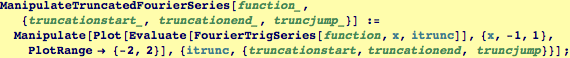Efficient Calculation of Truncated Fourier SeriesThe function above will work, but it is horribly inefficient!  Because it asks FourierTrigSeries to calculate one more term each time, it is doing some redundant work.  We can fix this up by having
it calculate one new term and adding to the sum calculated previously. Here it is:Created by Wolfram Mathematica 6.0  (24 October 2007)